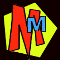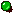#Squared Triangle and Cube numbers

#### 13 + 2 3 + 3 3 + 4 3 +....+ n 3 = (1 + 2 + 3 + 4 + ... + n)2

This ALWAYS works!#### TRIANGLE NUMBERS

If you arrange counters in triangular patterns, you get the triangle numbers. (The smallest "triangle" only needs one counter so it doesn't look much like a triangle.) To save time the "nth" triangle number is written as Tn. Here's what the first four triangles look like:If you wanted to work out the third triangle number without using counters you just put T3 = 1+2+3 =6.

Otherwise you can use the triangle number formula: Tn = n(n+1)/2

To work out T3 you use the formula making n=3.
You get Tn = 3(3+1)/2 = 3x4/2 = 12/2 = 6.

The secret is that (1 + 2 + 3 + 4 +... +n) makes the nth TRIANGLE NUMBER. Triangle numbers are explained in More Murderous Maths and Numbers, but all you need to know for now is in the black box with the groovy orange writing.

When you have (1 + 2 + 3 + ... n)2, then you are working out the nth triangle number squared. To make this easier to write we can put (Tn)2. Now we can make our mystery formula a bit neater:

13 + 2 3 + 3 3 + 4 3 +....+ n 3 = (Tn)2

Suppose instead of going up to number n we went up to the next number: (n+1). We'd get:

13 + 2 3 + 3 3 + 4 3 +....+ n 3+(n+1)3 = (T(n+1))2

Let's see how the two sides have changed. On the left hand side, we've just added on an extra (n+1)3. On the right hand side it's a bit more complicated. We had the nth triangle number squared, now we've got the (n+1)th triangle number squared. What we need to show is that the difference between (T(n+1))2 and (Tn)2 is (n+1)3.

The formula for Tn is in the box, and to get the formula for T(n+1) we just replace n with (n+1).
We get T(n+1) = {(n+1)(n+2)/2 }

Now when we work out (T(n+1))2 - (Tn)2 we get:

{(n+1)(n+2)/2 }2 - { n(n+1)/2 }2

= (n+1)2(n+2)2/4 - n2(n+1)2/4Both bits contain (n+1)2/4 so we can "factorise" - which means taking it out and putting it at the front.

= {(n+1)2/4} x {(n+2)2 - n2}

= {(n+1)2/4} x { n2 +4n +4 - n2}

= {(n+1)2/4} x {4n +4}

= {(n+1)2/4} x 4{n +1}

= (n+1)2 x (n+1)

= (n+1)3

That's the answer we wanted to see! We've shown that the difference between (T(n+1))2 and (Tn)2 is (n+1)3, and that's why our mystery formula always works!If you like this then you'll LOVE Cube Number Triangles!